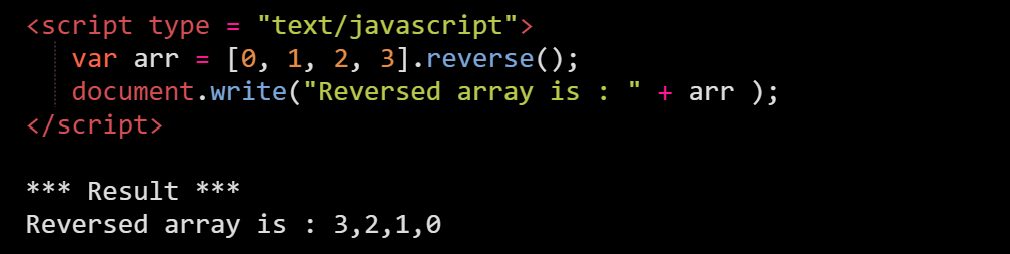# Methods

Methods represent some action we wish to perform on the array. They can be used to change the behaviour or perform some operations on itself.

To make things easier, arrays provide a lot of methods. Let’s go over some of the basic ones which would give you an idea of they look like.

# Concat()

Joins two or more arrays and returns a new array.

Syntax:

``````var value = arrayName1.concat(arrayName2);
``````

Example: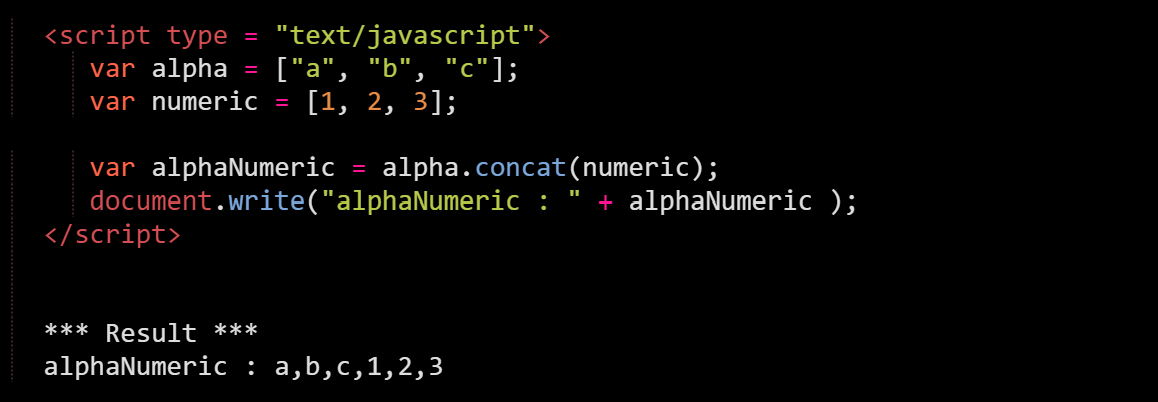# Push()

Adds one or more elements to the end of an array and returns the resulting length of the array.

Syntax:

``````var value = arrayName.push(newElement);
``````

Example: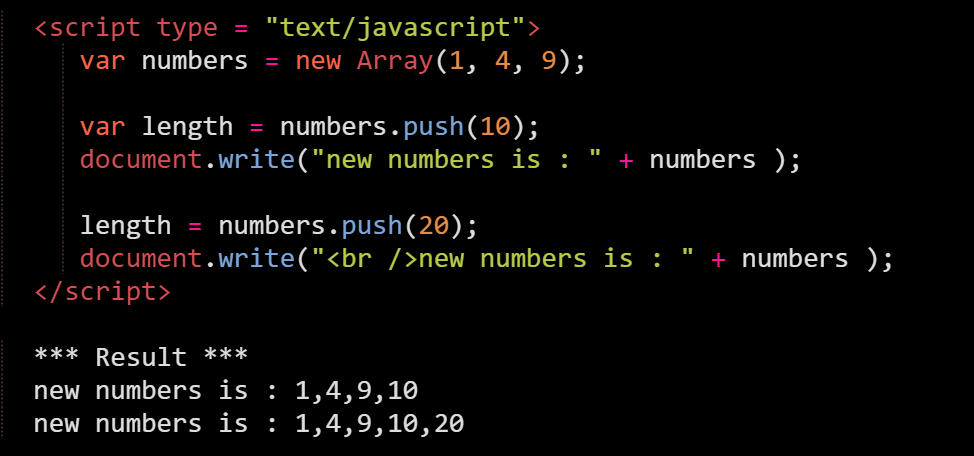# Pop()

Removes the last element from an array and returns that element.

Syntax:

``````var value = arrayName.pop();
``````

Example: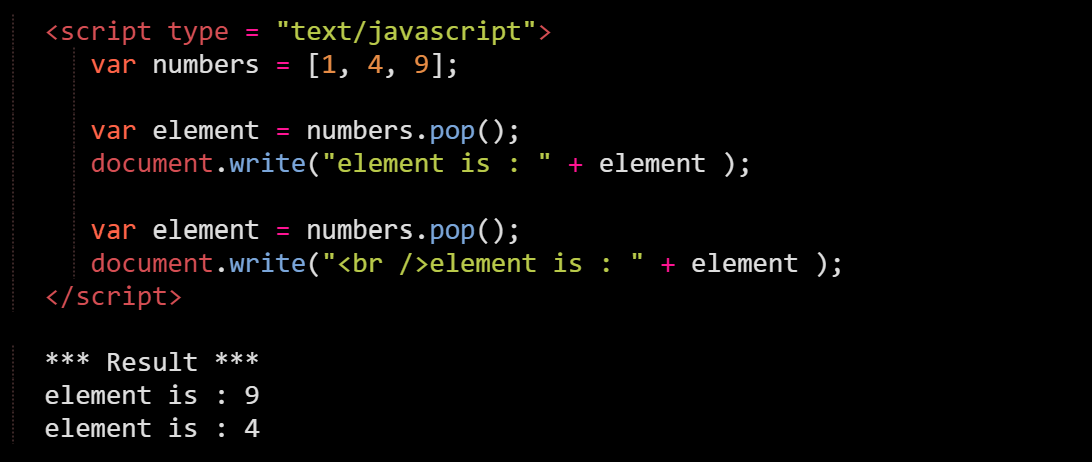# Shift()

Removes the first element from an array and returns that element.

Syntax:

``````var value = arrayName.shift();
``````

Example: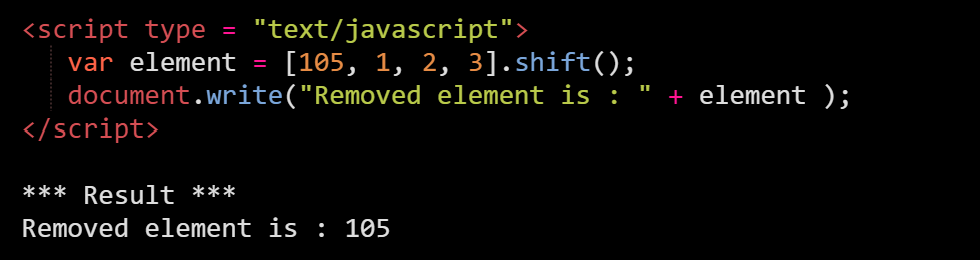# Unshift()

Adds one or more elements to the front of an array and returns the new length of the array.

Syntax:

``````var value = arrayName.unshift(newElement);
``````

Example: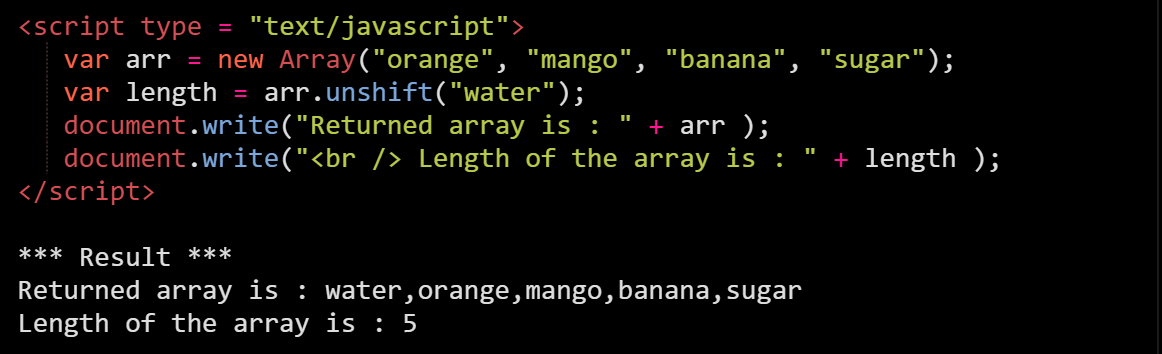# Sort()

Sorts the elements of an array in place, and returns a reference to the array.

Syntax:

``````var value = arrayName.sort();
``````

Example: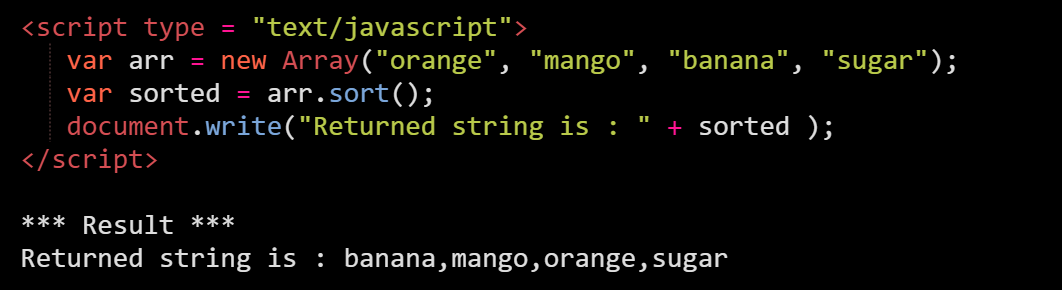# Reverse()

Transposes the elements of an array, in place: the first array element becomes the last and the last becomes the first. It returns a reference to the array.

Syntax:

``````var value = arrayName.reverse();
``````

Example: